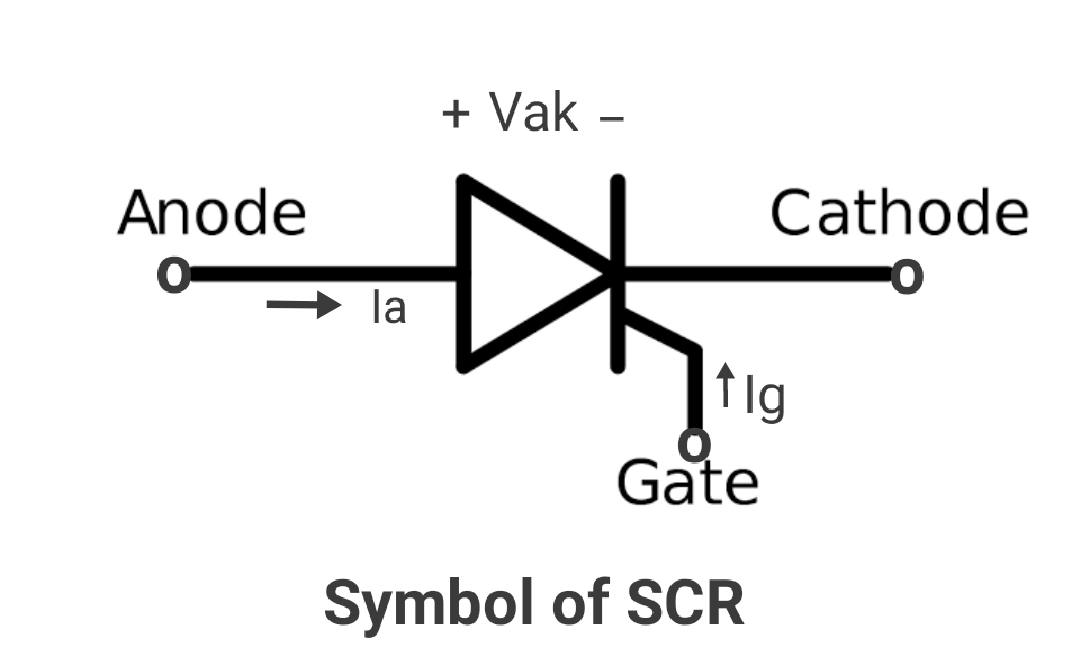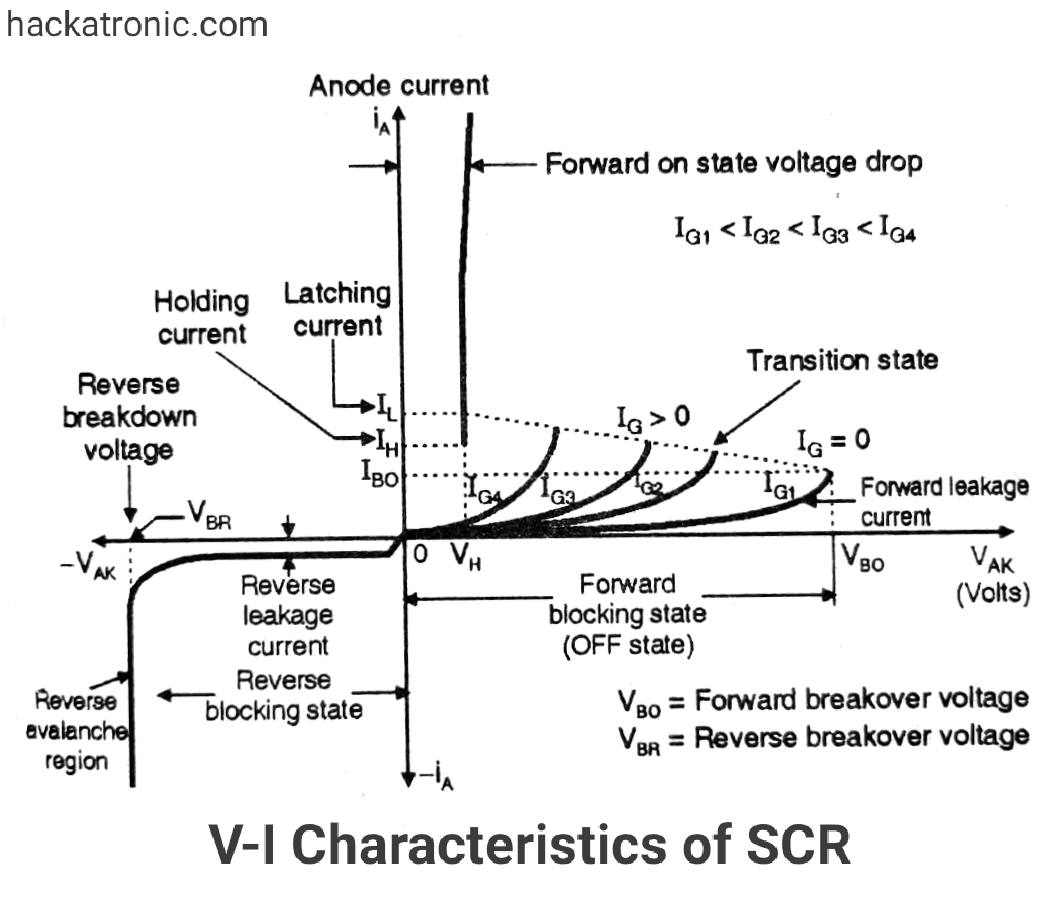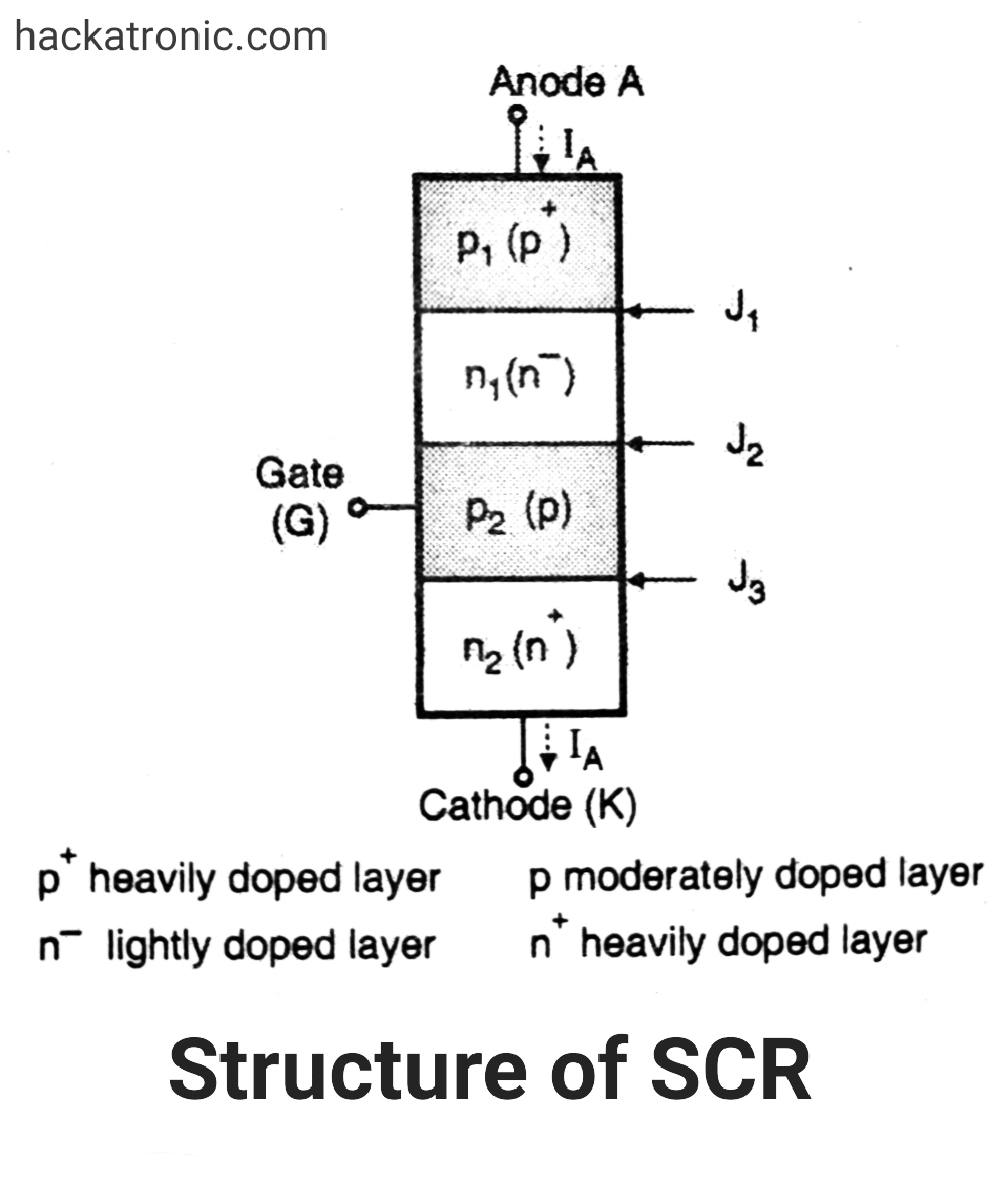# VI Characteristics of SCR (Silicon controlled rectifier)

The VI characteristics of SCR(silicon controlled rectifier) is a graph of anode current Ia on the y-axis and anode to cathode voltage on the x-axis as shown in the graph.

The characteristics in the reverse direction (anode to cathode voltage negative) is similar to a reverse-biased diode. SCR is a member of the thyristor family.

I will suggest you read the Working of silicon controlled rectifier SCR. also Applications of SCR.## VI characteristic of SCR:

The VI Characteristics of SCR can be split into two parts namely

• Forward characteristics
• Reverse characteristics

For small reverse voltage, a small reverse leakage current flows. until the avalanche breakdown takes place at reverse breakover voltage Vbr.

As soon as the reverse breakdown takes place due to avalanche breakdown, a large current flows through SCR whereas the voltage across the device remains constant.It is dangerous to operate SCR in the reverse breakdown state because it may get damaged due to overheating.

The region from 0 Volts up to Vbr volts in which the SCR is reverse biased and non-conducting is called as “reverse blocking state”. Reverse blocking means that the SCR is reverse biased and in the non conducting (blocking) state.

### Forward characteristics of SCR:

The anode is positive with respect to the cathode. Hence SCR is forward biased.

The forward characteristics are divided into three regions of operation namely:

1. Forward blocking state.
2. Transition state.
3. ON state.#### Forward blocking state :

This is the high voltage low current mode of operation in which SCR is in the OFF state. The current through it is “Forward Leakage Current” This current flows due to thermally generated minority carriers.

#### Transition state :

SCR remains in the blocking state as long as the forward anode to cathode voltage is less than the breakover voltage.

As soon as Vak becomes greater than the breakover value, and the anode current Ia increases sharply to a high value and the voltage across SCR reduces sharply to a low value (on-state voltage).

The switching of SCR from off state to on state and vice versa takes place in a short time. This changeover state is called as transition state. As this is an unstable state, it is shown by the dotted line.

#### ON state:

The voltage across SCR is low in this state. In the on-state the SCR anode currents is large and the on-state voltage drop is small.

#### Unstable state :

These two stable states or modes are connected by an unstable mode (called as transition state) of operation that appears as a negative resistance on the I-V characteristics.

### The important terms in the IV characteristics are as follows:

Here are the most important terms in the VI Characteristics of SCR.

#### The forward breakover voltage (Vbo):

This is the maximum forward voltage that can be applied between anode and cathode, without initiating forward conduction. This voltage is defined for a zero gate current. In short, this is the maximum forward voltage across SCR in its off state.

#### The forward leakage current:

The small forward current flowing in the forward blocking state of the device. This current is generated due to the minority current carriers and therefore is dependent on the operating temperature.

#### The holding current (Ih):

It represents the minimum current that can flow through SCR and still “hold” it in the on state. The voltage associated with the holding current is termed as holding voltage Vh.

If the forward anode current is reduced below holding current, SCR will be turned off. The holding current is defined for zero gate current (Ig = 0), it is denoted by Ih and its value is few mA.

#### ON-state voltage:

Note that the voltage across SCR in its on state is very low as compared to the off-state voltage. It can be shown that the on-state voltage is equal to drop across one junction (1 to 1.5 Volts).

#### Latching current (il):

It is the minimum anode current that must flow through SCR to latch it into the on-state. The latching current is higher than the holding current. The latching current is important when SCR is being turned on.

### Difference between holding current and latching currents:

1. The latching current is important only at the time of SCR turn on, whereas holding current is important only at the time of SCR turn off.
2. If the anode current goes below the holding current then the conducting SCR turns off. The holding current is defined for If = 0, whereas if anode current at the time of turn-on is higher than the latching current then only SCR will latch into its on state.
3. If Ia < IL then SCR does not latch. It will remain on as long as Ig is being supplied. As soon as Ig is removed, SCR will turn off.
4. The latching current is always higher than the holding current.
5. The values of IL and Ih are constant. They do not depend on the gate current magnitude.

#### Effect of gate current :

The graph shows that as we increase the positive gate current from Ig1 to Ig4, the breakover voltage of SCR Vbo reduces progressively. Thus SCR will turn on at lower anode to cathode voltages as we increase the gate current.

### Reverse Characteristics of SCR:

The reverse characteristics of an SCR is very similar to that of a diode.

As long as the reverse anode to cathode voltage is less than the reverse breakover voltage, a small reverse current flows due to minority carriers.

But as soon as a reverse voltage equal to or greater than breakdown voltage is applied, a very large anode current flows due to avalanche breakdown. This should be avoided to protect the SCR from getting damaged.

This site uses Akismet to reduce spam. Learn how your comment data is processed.